# RBI Grade B Phase 1 Quant Quiz 2023 – 3 March

Directions (1-5): Study the information carefully and answer the questions given below.
A, B, C, D and E have 5 friends. They work together in different positions in the office. Salary they spend only on food and rent. (1) The money spent by A on food is the same as the money spent by B on rent. The money spent by C and E on food and rent is in the ratio 3 : 5 and 2 : 3 respectively. The money spent on rent by A, C and D is in the ratio 3:2:1 and the money spent on food by B and D is in the ratio 3:7.
(2) Salaries of A, B, D and E are in the ratio 11:8:9:10 and E’s salary is Rs.50000 out of Rs.222000.

Q1. Find the ratio of the salaries of C and A to B and D together.

(a) 87:85
(b) 85:73
(c85:87
(d) 23:31
(e) 41:1

Q2. The amount spent by C on rent is what percentage of the amount spent by D on rent?

(a) 20%
(b) 200%
(c) 22%
(d) 11%
(e) 5%

Q3. How much more is the amount spent by A, B and C on food than the amount spent by D and E on rent? (in Rs.)

(a) 10000
(b) 11000
(c) 12000
(d) 30000
(e) 11500

Q4. What is the difference (in Rs) between the average salary of B and C and the combined average salary of D and E?

(a) 21230
(b23456
(c) 12345
(d) 11500
(e) 55110

Q5. Find 50% of the total money spent on rent and 30% of the total money spent on food (in Rs).

(a) 11230
(b) 33200
(c) 34000
(d) 89900
(e) 89600

Directions (6-10): The following pie-chart shows the percentage of candidates who passed the RBI examination from cities A, B, C, D, E and F out of the total number of candidates who passed from six cities in 2010. And the line graph shows the percentage of freshers. Candidates passed in each city out of the total number of pass candidates in that city in 2010. Study the data carefully and answer the questions.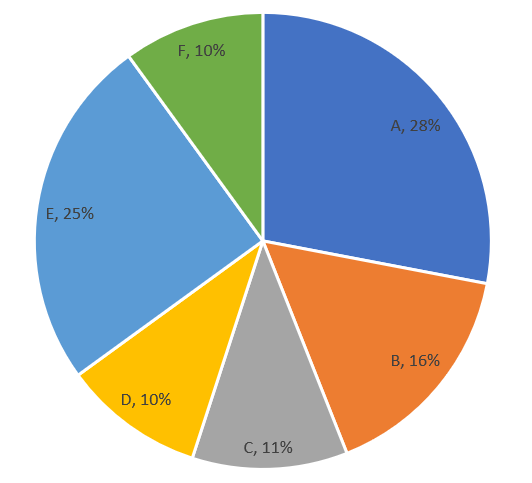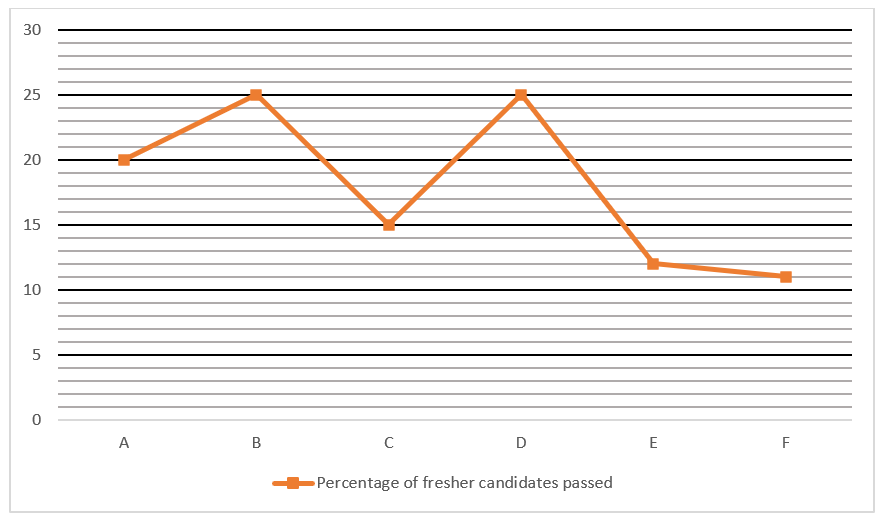Q6. If in 2010, the total number of freshers passed from city D was 320, how many freshers candidates from city E passed the RBI exam?

(a) 384
(b) 284
(c) 364
(d) 360
(e) 344

Q7. If in 2010, the total number of passed candidates from all cities was 1250, what is the number of non-fresher candidates from city A who passed RBI?

(a) 140
(b) 210
(c) 420
(d) 280
(e) 320

Q8. If the number of failed candidates from city B was 180 in 2010, then how many total candidates from all the cities passed the RBI exam?

(a) 1450
(b) 1200
(c) 1500
(d) 1250
(e) 1800

Q9.The number of passed candidates from city A and B increased by 10% and 20% respectively in 2011 as compared to 2010 and the number of passed candidates from city C was 770 in 2010. Then what will be the difference in the number of candidates passed from city A and B in 2011?

(a) 712
(b) 812
(c) 912
(d) 880
(E) 822

Q10. If the total number of pass candidates from city B in 2010 is 320, what is the ratio of the number of new pass students from city A to the number of pass students from city C?

(A) 112 : 187
(B) 113 : 186
(c) 115 : 189
(d) 187 : 112
(e) None of these

Directions (11-15): Study the given data carefully and answer the questions based on it.
Three shops sold two different types of computer mice (wired + wireless). The number of wired computer mice sold by X is 240. The ratio between the number of wireless computer mice and the number of wired computer mice sold by X is 5 : 3. total number. The number of wireless computer mice sold by all stores is 960. The total number of computer mice sold by store Y is 25% more than the total number of computer mice sold by store X. The number of wireless computer mice sold by store X is 80% of the number of wired computer mice sold by store Y. The average number of total computer mice sold across the three stores is 690.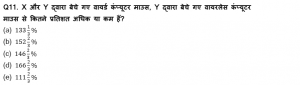Q12. Find the ratio of the total computer mice sold by Z to the total wired computer mice sold by all three stores.

(A) 21 : 37
(b) 17:31
(c) 19:29
(d) 21:31
(E) 23:39

Q13. If the number of wireless computer mice sold by store A is 40% more than the number of wired computer mice sold by store X and the average number of computer mice sold by X and A is 637, then find the number of wired computer mice sold by store A

(a) 271
(b) 308
(c) 278
(d) 298
(E) 288

Q14. A wired computer mouse costs 2/3 of the price of a wireless computer mouse. If Store X made a profit of Rs 67200 by selling all the computer mice, find the price of the wireless computer mouse.

(a) Rs.80
(b) Rs. 120
(c) Rs. 160
(d) Rs. 100
(e) Rs. 125

Q15. If 30% of the total computer mice sold by store Z are defective and the ratio of defective wired computer mouse to defective wireless computer mouse is 5 : 4, find the difference between non-defective wired computer mouse and non-defective wireless computer mouse.

(a) 76
(b) 93
(c) 102
(d) 81
(E) 89

Solution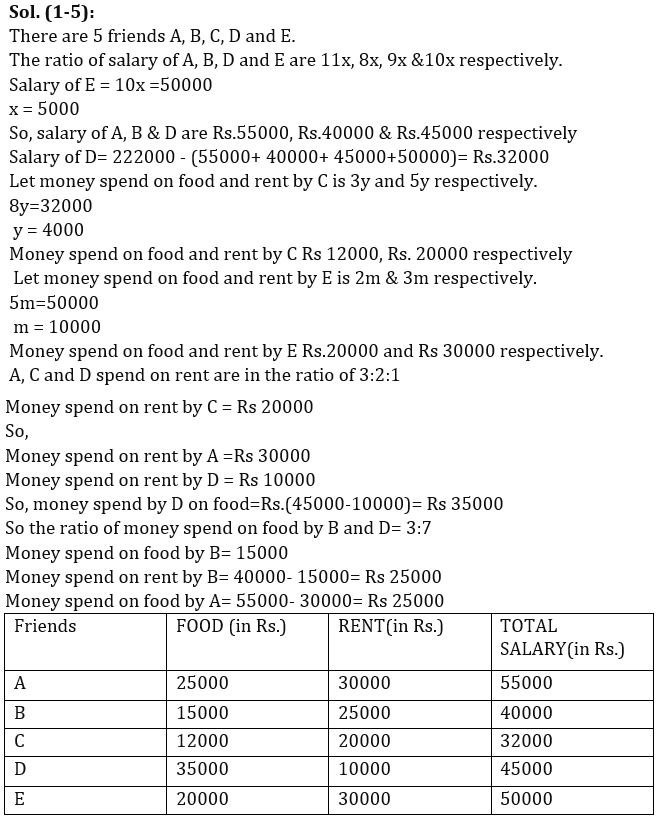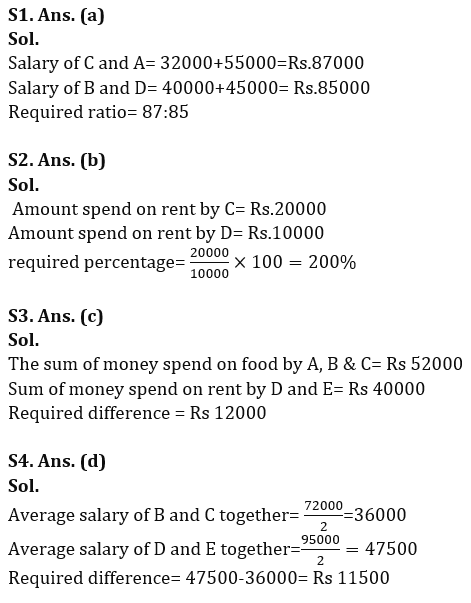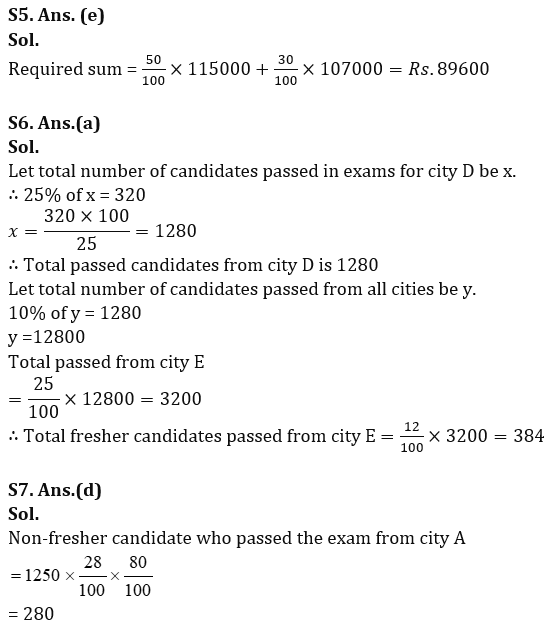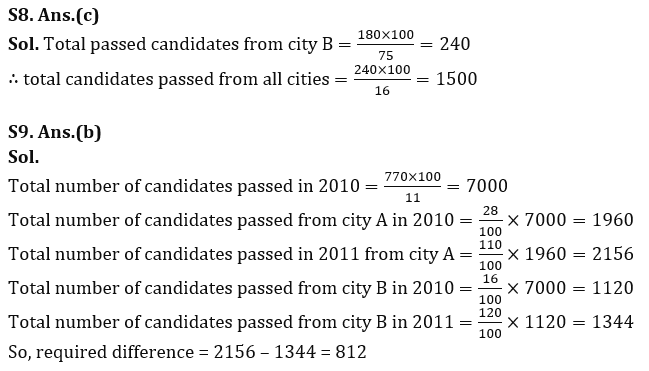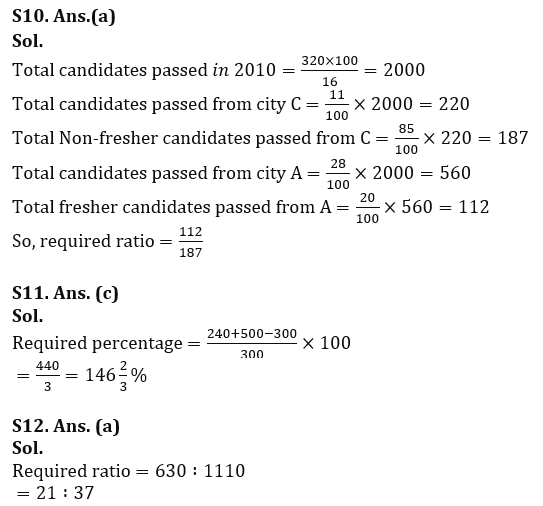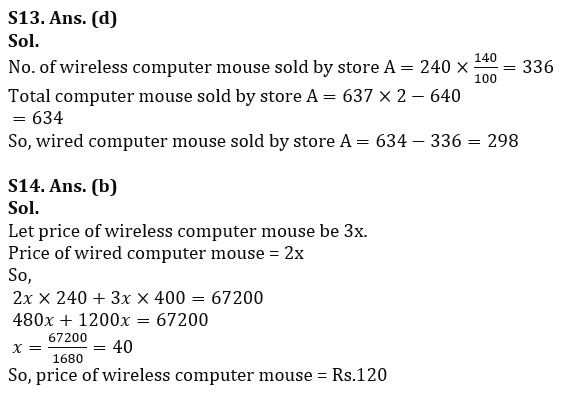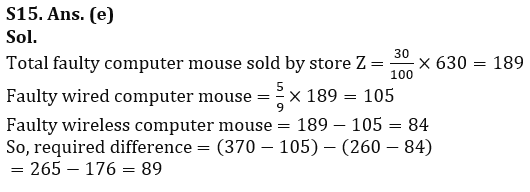,